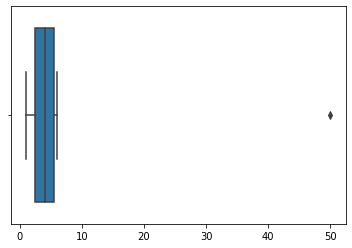# Interquartile Range to Detect Outliers in Data

An observation which differs from an overall pattern on a sample dataset is called an outlier.

Outliers:
The outliers may suggest experimental errors, variability in a measurement, or an anomaly. The age of a person may wrongly be recorded as 200 rather than 20 Years. Such an outlier should definitely be discarded from the dataset.
However, not all outliers are bad. Some outliers signify that data is significantly different from others. For example, it may indicate an anomaly like bank fraud or a rare disease.

Significance of outliers:

• Outliers badly affect mean and standard deviation of the dataset. These may statistically give erroneous results.
• Most machine learning algorithms do not work well in the presence of outlier. So it is desirable to detect and remove outliers.
• Outliers are highly useful in anomaly detection like fraud detection where the fraud transactions are very different from normal transactions.

What is Interquartile Range IQR?

IQR is used to measure variability by dividing a data set into quartiles. The data is sorted in ascending order and split into 4 equal parts. Q1, Q2, Q3 called first, second and third quartiles are the values which separate the 4 equal parts.

• Q1 represents the 25th percentile of the data.
• Q2 represents the 50th percentile of the data.
• Q3 represents the 75th percentile of the data.

If a dataset has 2n / 2n+1 data points, then
Q1 = median of the dataset.
Q2 = median of n smallest data points.
Q3 = median of n highest data points.

IQR is the range between the first and the third quartiles namely Q1 and Q3: IQR = Q3 – Q1. The data points which fall below Q1 – 1.5 IQR or above Q3 + 1.5 IQR are outliers.

Example:
Assume the data 6, 2, 1, 5, 4, 3, 50. If these values represent the number of chapatis eaten in lunch, then 50 is clearly an outlier.
Step by step way to detect outlier in this dataset using Python:

Step 1: Import necessary libraries.

 `import` `numpy as np  ` `import` `seaborn as sns `

Step 2: Take the data and sort it in ascending order.

 `data ``=` `[``6``, ``2``, ``3``, ``4``, ``5``, ``1``, ``50``] ` `sort_data ``=` `np.sort(data) ` `sort_data `

Output:

`array([ 1,  2,  3,  4,  5,  6, 50])`

Step 3: Calculate Q1, Q2, Q3 and IQR.

 `Q1 ``=` `np.percentile(data, ``25``, interpolation ``=` `'midpoint'``)  ` `Q2 ``=` `np.percentile(data, ``50``, interpolation ``=` `'midpoint'``)  ` `Q3 ``=` `np.percentile(data, ``75``, interpolation ``=` `'midpoint'``)  ` ` `  `print``(``'Q1 25 percentile of the given data is, '``, Q1) ` `print``(``'Q1 50 percentile of the given data is, '``, Q2) ` `print``(``'Q1 75 percentile of the given data is, '``, Q3) ` ` `  `IQR ``=` `Q3 ``-` `Q1  ` `print``(``'Interquartile range is'``, IQR) `

Output:

```Q1 25 percentile of the given data is, 2.5
Q1 50 percentile of the given data is, 4.0
Q1 75 percentile of the given data is, 5.5
Interquartile range is 3.0```

Step 4: Find the lower and upper limits as Q1 – 1.5 IQR and Q3 + 1.5 IQR, respectively.

 `low_lim ``=` `Q1 ``-` `1.5` `*` `IQR ` `up_lim ``=` `Q3 ``+` `1.5` `*` `IQR ` `print``(``'low_limit is'``, low_lim) ` `print``(``'up_limit is'``, up_lim) `

Output:

```low_limit is -2.0
up_limit is 10.0```

Step 5: Data points greater than the upper limit or less than the lower limit are outliers

 `outlier ``=``[] ` `for` `x ``in` `data: ` `    ``if` `((x> up_lim) ``or` `(x

Output:

` outlier in the dataset is `

Step 6: Plot the box plot to highlight outliers.

 `sns.boxplot(data) `Step 7: Following code can also be used to calculate IQR

 `from` `scipy ``import` `stats ` `IQR ``=` `stats.iqr(data, interpolation ``=` `'midpoint'``) ` `IQR `

Output:

`3.0`

Conclusion: IQR and box plot are effective techniques to detect outliers in data.

My Personal Notes arrow_drop_upCheck out this Author's contributed articles.

If you like GeeksforGeeks and would like to contribute, you can also write an article using contribute.geeksforgeeks.org or mail your article to contribute@geeksforgeeks.org. See your article appearing on the GeeksforGeeks main page and help other Geeks.

Please Improve this article if you find anything incorrect by clicking on the "Improve Article" button below.